# The tutor continues with algebra tiles. Tutoring math, you see them especially in grades 9 or 10.

I began discussing algebra tiles here. Recall that they are meant to model numbers, variables, and operations you can perform to them. Recalling the foil method, we’ll take a look at how algebra tiles can be used to “show” it.

Example: Show (x+6)(x-2) with algebra tiles.

Solution: We know by the foil method that the answer is x^2+4x-12.

With algebra tiles, the layout is rows and columns. We might, for instance, display x+6 across the top, and x-2 down the left, as follows:The result of the multiplication is in the “body” of the table. We see one large white square (which means +x^2), six white rectangles (+6x), then two black rectangles (-2x), and finally, twelve small black squares (-12). The two black rectangles cancel out two of the white ones, leaving four. The result is x^2 +4x -12, as we knew from foil.

Some people find foil quite difficult, while this visual method is easier for them. Once they’re used to it, they hopefully become comfortable with how it translates to foil.

I’ll be saying more about algebra tiles in future posts:)

Jack of Oracle Tutoring by Jack and Diane, Campbell River, BC.

Tagged with: ,

# Tutoring high school math, radicals are prominent.  The math tutor introduces addition and subtraction of them.

Adding radicals is much like adding variables. Note that x=1x; the 1 is understood to be there, but never written. Similarly, √3=1√3. Therefore, 2√5 + √5 = 3√5

You can’t simplify x plus y; x+y is just x+y. Similarly, √6+√7 doesn’t simplify. A calculator will give you a decimal for it, but that’s not an exact value.

So what about the following:

Example 1: Simplify √(12)+√(75)+√3

You may want to read up on simplifying radicals in my earlier post to understand what follows.

Solution: On the face of it, we can’t add the radicals because they’re not the same kind. However, we can simplify some of them as follows:

√(12) = 2√3

√(75) = 5√3

Simplifying reveals them to be the same kind. We indeed can add them.

√(12)+√(75)+√3 becomes

2√3 + 5√3 + √3 = 8√3

By a similar principle, √(50)-√8=5√2-2√2=3√2

I’ll be covering some different examples in an upcoming post:)

Jack of Oracle Tutoring by Jack and Diane, Campbell River, BC.

Tagged with: , ,

# When you tutor high school chemistry, you cover a little organic chemistry. Today we visit a topic that rarely gets asked about during tutoring, but is very useful to know at the college level.

In my previous post on organic chemistry, I introduced the topic and showed a few drawings. They were of this style: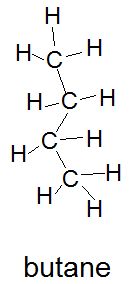Recall that carbon, which appears as C in the diagrams, makes four bonds. (Each bond is a line to another atom.) Notice that most of the bonds are between carbon and hydrogen (H).

Since bonds usually are between C and H, chemists often don’t draw the H atoms. They’re understood to be there unless a bond clearly goes to something else. In such cases, only the bonds between carbons or between carbons and other atoms are shown, like so: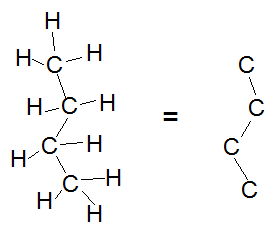With the hydrogens left out, the diagram becomes simpler to understand. For example, you can more easily tell the how many carbons it has.

Some people take simplification a step further, writing only sticks for the carbon skeleton. Each corner represents a carbon atom. Therefore, all three of the following diagrams show butane: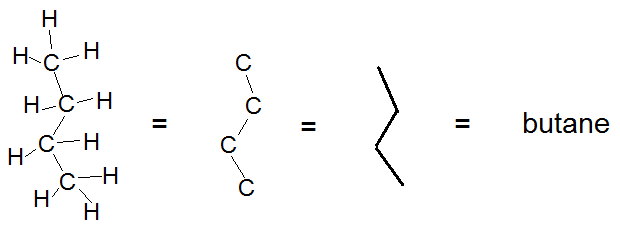The simplified drawing styles really come in handy for showing molecules with features such as alcohol. Here’s an example:How we know the molecule is 2-pentanol will be covered in a future post:)

Jack of Oracle Tutoring by Jack and Diane, Campbell River, BC.

Tagged with: ,

# Tutoring math, you might explain algebra tiles a few times a year.  The math tutor introduces them.

If algebra tiles existed when I was in school, I never saw them. Everything back then was symbolic, and teaching didn’t have the directive of making the learning easier.

Nowadays, attitudes towards teaching and learning couldn’t be more different. Manipulatives, as they are called, are used to explain counting and even variables in a visual, physical way. An abacus could be used thus.

Algebra tiles are used to depict expressions with variables. A big square means x^2, a rectangle means x, and a small square means 1. Consider the following example:

Example 1: Show 3x^2 + 4x +5 with algebra tiles.

Solution: We need three big squares for the 3x^2, then four rectangles to make 4x, and finally five small squares to indicate 5. Like so:Depending on the situation, you may need to show the rectangles horizontally and/or group the small squares in various different ways. Here is another depiction of 3x^2 + 4x +5:How do you show negative values? With color, is the answer. Often, the light coloured tiles are taken to mean positive, while the darker ones mean negative.

Example 2: Show -2x^2 -x + 3 with algebra tiles.That’s a pretty good beginning with algebra tiles. In future posts I’ll show some of the math concepts you can illustrate with them:)

Jack of Oracle Tutoring by Jack and Diane, Campbell River, BC.

Tagged with:

# Tutoring English, a constant question is how to keep writing fresh.  The English tutor discusses his recent satisfaction with the word while….

Continuing, generally, the discussion about complex sentences that began with my last post, I’d like to tell you about my rediscovery of the word while.

Last summer, I had several writing projects to complete. Needing, as always, to maintain sentence variety, yet without unnecessary length, I gradually zeroed in on while. I never used it much in high school or university, but now I’m finding new uses for it weekly.

While is a great word: I think the reason is that it has two potential meanings. It can mean “at the same time” or “although.” Often, those two meanings apply simultaneously.

Consider the following example:

While he hated math, his highest mark was in it.

For a situation to be ironic, two conflicting premises need to be true at the same time. That’s why while, I’m finding, shines among subordinating conjunctions.

While India’s economic growth rate may lag China’s some years, India is ahead in being a democracy.

In one of my writing endeavours last summer, the target was really stingy with word allowance. Being a single word with two potential meanings, while came to my rescue time and again. Maybe it will for you, too:)

Jack of Oracle Tutoring by Jack and Diane, Campbell River, BC.

Tagged with: , ,

# Tutoring high school English, sentence variety is a constant concern.  It’s the same situation for college students and writers.  The English tutor shares one commonly known – and appreciated – type of sentence.

Definitely, one preferred structure in high school English is what I’d call the complex sentence.  It has two parts: one dependent, one independent.  (The independent part can stand alone as a sentence, while the dependent part cannot.)  Let’s look at an example:

Because she set the burner too high, the eggs are rubbery.

In the sentence above, Because she set the burner too high is the dependent part (aka, dependent clause). It’s clearly a reason, but doesn’t make sense unless you know what for. The independent part (clause) is the eggs are rubbery . “The eggs are rubbery” has a subject and an action; it’s a standalone sentence. It’s not dependent on another idea because it doesn’t have a subordinating conjunction.

The subordinating conjunction in the sentence above is because. It makes she set the burner too high a reason for the other premise – that the eggs are rubbery. In a complex sentence, the subordinate – aka, dependent – clause exists to somehow serve the independent one. By “serve”, we mean give it more meaning. The subordinate clause in the example above explains the reason behind the independent one. However, the subordinate clause can relate to the independent one in other ways as well. For instance, the subordinate clause often expresses surprise or irony, as in the next example:

The ball game played as scheduled, although it was a rainy evening.

Once again, the dependent – or subordinate – clause is typed in green. In this example, the subordinating conjunction is although. It expresses surprise that, even with the rain, the game went on.

There are many common subordinating conjunctions: after, although, because, before, once, since, unless, while…., just to name a few.

When written correctly, complex sentences are generally appreciated by teachers as showing an advanced level of writing. Therefore, they’re worth understanding and getting right.

Now that I’ve started this thread, I’ll be talking more about it soon. Until then, enjoy this spectacular spring day!

Source: Hodges, Webb, Miller, Stubbs: Harbrace Handbook for Canadians.                              Scarborough: Nelson Education Ltd., 2003.

Jack of Oracle Tutoring by Jack and Diane, Campbell River, BC.

# Tutoring math 12, you may discuss counting problems.  The tutor offers a quick guide for when to multiply vs when to add.

One question you’re sometimes asked about counting is when you multiply, vs when you add.  The answer is simple.  Consider two events, one of which can happen n ways, while the other can happen m ways.

If the events are both going to happen, then the total number of different ways they can happen is nm; you multiply.  However, if only one of the events will happen, but it could be either one, then there are n + m outcomes; you add in that case.  Let’s consider a couple of examples:

Example 1

Bill is going to order dinner and dessert.  There are five dinner choices and three dessert choices.  How many ways can he order the two courses?

Solution:  We know he will order both, so we multiply to get the total number of possibilities.  He can order the two courses in 5×3 = 15 different ways.

Example 2

On a different night, Bill finds himself at another restaurant.  Being in a hurry, he will just order dinner.  There are seven meat choices and three vegetarian choices.  Looking at the menu, Bill is not yet sure whether he will go meat or vegetarian tonight.  How many possible orders could he give for dinner?

Solution:  Bill will only order one dinner.  However, he could go either meat or vegetarian.  Since we don’t know which, we must add the possibilities to get the total. He could order dinner in 7+3 10 different ways.

These counting rules are simple, yet they clarify a good many problems that might seem harder at first.  Good luck with your counting problems:)

Jack of Oracle Tutoring by Jack and Diane, Campbell River, BC.

Tagged with:

# Tutoring math, you know that div and mod are also fundamental to computer science.  The tutor presents them with an example.

Consider the age-old long division situation: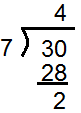In this case, the question posed is “30 divided by 7”. The answer is “4, remainder 2.” But in terms of div and mod, what does it mean?

4 is the answer to 30 div 7. The div operation gives the integer quotient of a division: it gives the number of times the divisor can go in. The mod operator, on the other hand, gives the remainder of a division: 30 mod 7 is 2, just as shown above.

A really neat example of div and mod in action is the following:

Example: Convert 103 inches to feet and inches

Solution: Set it up long division style, like so: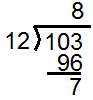The answer is that 103 inches is 8 feet, 7 inches. 103 div 12=8 gives the feet, while 103 mod 12=7 gives the inches. Once again: div gives the integer quotient, whereas mod gives the remainder.

For more implications of div and mod, come visit again:)

Jack of Oracle Tutoring by Jack and Diane, Campbell River, BC.

# Tutoring math, you cover this topic with students in late middle school or early high school.  The math tutor shows the first case.

This article assumes that the reader is familiar with multiplying radicals. If necessary, see my article here about that.

Rationalizing the denominator is done when there’s a radical on the bottom of a fraction.  Consider the following:

Example 1: Simplify 7/(2√(3))

Solution:

To rationalize the denominator, you multiply the top and bottom by the same number. The number is chosen so it turns the radical on the bottom into a whole number:

7/(2√(3))*√(3)/√(3)=7√(3)/(2√(3)√(3))

Above, we have multiplied 7/(2√(3)), top and bottom, by √(3). We can do so because when you multiply the top and bottom by the same amount, the fraction’s value doesn’t change, just its form. Notice that √(3)√(3)=3. Therefore,

7/(2√(3))*√(3)/√(3)=7√(3)/(2√(3)√(3))=7√(3)/(2*3)=7√(3)/6

So it turns out that, when you rationalize the denominator,7/(2√(3)) becomes 7√(3)/6

Of course, there are more complicated situations where you have to rationalize the denominator. I’ll get to them in future posts:)

Jack of Oracle Tutoring by Jack and Diane, Campbell River, BC.

Tagged with: ,

# Tutoring high school math, you realize that radicals are a problem for many. Today the math tutor gives brief coverage of multiplying radicals.

Consider the following example:

Simplify 3√(6)*4√(7)

Multiplying with radicals goes “number times number, radical times radical.” Like so:

Step 1: Number times number: In our case, 3 times 4, which gives 12.

Step 2: Radical times radical. In our case, √(6)√(7) gives √(42).

Putting it all together, we get

3√(6)*4√(7)=12√(42)

So far, so good. Beware, though: a simplification can creep in when the radicals are multiplied together:

Example 2: Simplify 3√(10)*7√(6)

Solution: Using number times number, radical times radical, we get

21√(60)

From my earlier post on simplifying radicals, we know we can’t just leave the answer 21√(60). After all, 60 contains the perfect square 4; we must simplify it as follows:

21√(60)=21√(4)√(15)=21*2√(15)=42√(15)

Note that if one of the factors is missing the number part, you just imagine it’s a 1 and proceed:

4√(7)*√(11)=4√(7)*1√(11)=4√(77)

On the other hand, one of the factors might be missing the radical part. Then, you just multiply the numbers together and tack the radical at the end:

6√(5)*(8)=48√(5)

Visit again for more tips on operations with radicals:)

Jack of Oracle Tutoring by Jack and Diane, Campbell River, BC.

Tagged with: , ,
Top